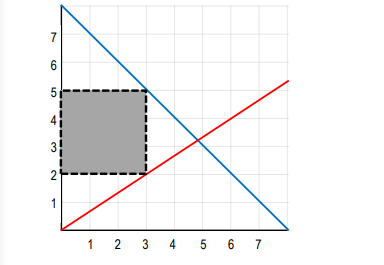Ch. 1 - Introduction to MicroeconomicsWorksheetSee all chapters
 Ch. 1 - Introduction to Microeconomics 1hr & 57mins 0% complete WorksheetDownload the video lesson worksheet Ch. 2 - Introductory Economic Models 1hr & 30mins 0% complete WorksheetDownload the video lesson worksheet Ch. 3 - Supply and Demand 3hrs & 49mins 0% complete WorksheetDownload the video lesson worksheet Ch. 4 - Elasticity 2hrs & 31mins 0% complete WorksheetDownload the video lesson worksheet Ch. 5 - Consumer and Producer Surplus; Price Ceilings and Floors 3hrs & 45mins 0% complete WorksheetDownload the video lesson worksheet Ch. 6 - Introduction to Taxes and Subsidies 1hr & 47mins 0% complete WorksheetDownload the video lesson worksheet Ch. 7 - Externalities 1hr & 21mins 0% complete WorksheetDownload the video lesson worksheet Ch. 8 - The Types of Goods 1hr & 13mins 0% complete WorksheetDownload the video lesson worksheet Ch. 9 - International Trade 1hr & 27mins 0% complete WorksheetDownload the video lesson worksheet Ch. 10 - The Costs of Production 2hrs & 46mins 0% complete WorksheetDownload the video lesson worksheet Ch. 11 - Perfect Competition 2hrs & 24mins 0% complete WorksheetDownload the video lesson worksheet Ch. 12 - Monopoly 2hrs & 13mins 0% complete WorksheetDownload the video lesson worksheet Ch. 13 - Monopolistic Competition 1hr & 11mins 0% complete WorksheetDownload the video lesson worksheet Ch. 14 - Oligopoly 1hr & 26mins 0% complete WorksheetDownload the video lesson worksheet Ch. 15 - Markets for the Factors of Production 1hr & 34mins 0% complete WorksheetDownload the video lesson worksheet Ch. 16 - Income Inequality and Poverty 36mins 0% complete WorksheetDownload the video lesson worksheet Ch. 17 - Asymmetric Information, Voting, and Public Choice 39mins 0% complete WorksheetDownload the video lesson worksheet Ch. 18 - Consumer Choice and Behavioral Economics 1hr & 16mins 0% complete WorksheetDownload the video lesson worksheet

# Graphing Review

See all sections
Sections
Introduction to Economics
Three Key Economic Ideas
Productive and Allocative Efficiency; Equality
Factors of Production
Positive and Normative Statements
Circular Flow Diagram
Graphing Review
Percentage and Decimal Review
Fractions Review
###### Are you drowning in graphs? Let's review the skills you need to pass this class!

Concept #1: Plotting Points on a Graph

Concept #2: Drawing and Shifting Curves

Concept #3: Calculating Slope of a Straight Line

Concept #4: Calculating Slope of a Curve: Point Method

Concept #5: Calculating Slope of a Curve: Arc Method

Concept #6: Finding Maximum and Minimum on a Graph

Concept #7: Calculating Area of a Triangle on Graph

Concept #8: Calculating Area of a Rectangle on Graph

Practice: Calculate the area of the shaded region.Concept #9: Interpreting Graphs (Part One)

Concept #10: Interpreting Graphs (Part Two)